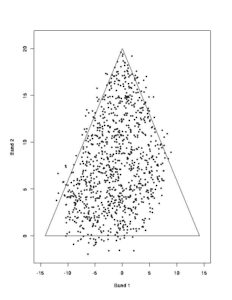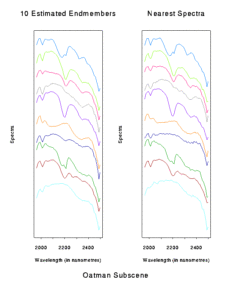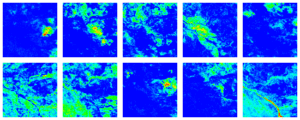# Current Research In Hyperspectral Imaging

#### How are These Problems Currently Addressed?

In recent years, a number of researchers have attempted to build “scene-specific” libraries to address the problem of incompatibility between ground based libraries and spectra captured from the air. The algorithms developed have attempted to find the “purest” pixels in an image or “scene”. These purest pixels are often called “endmembers”. This process is sometimes called unsupervised mixture decomposition and can be thought of as an extension of clustering. Most of the methods are based on a convex geometry model, which says that, if a pixel consists of certain materials, then the spectrum recorded at that pixel is a weighted sum or linear combination of the spectra of the constituent materials in the pixel, and that the weights are equal to the area proportions of each of the materials in that pixel. If the convex geometry model is true, and if there are M materials in a scene, then all the data in the scene lie inside a “simplex” with M vertices in some M – 1 dimensional subspace (e.g. if M = 3, the data lie inside a triangle in some two-dimensional subspace, while if M = 4, the data lie inside a pyramid or tetrahedron in some three-dimensional subspace). Hence, if there are, say, 10 distinct materials in the Oatman scene, the 54-dimensional data in Figs. 1 (a) and (b) can be compressed into a 9-dimensional subspace inside a simplex with 10 vertices, corresponding to the endmembers.

Fig. 1: (a) 54 AVIRIS shortwave infrared images of Oatman, Arizona (courtesy of NASA JPL). (b) “Stackplot” of spectra at 6 pixels in the Oatman Image. Please click on the images for an enlarged view.

Fig. 2 illustrates this concept with a toy example, where M = 3. The vertices of the triangle represent the 3 endmembers. We have simulated the observations at 1000 pixels in a scene. Each is some convex mixture of the endmembers. The example illustrates some real-world complications. First, the convex geometry model doesn’t hold exactly. There is noise in the data, due to (i) instrumental noise, (ii) natural variability in the spectra of materials that are nominally the same, and (iii) slight non-linearities in the mixing. So some observations lie a little outside the simplex. Second, not all the vertices are present in the data. For instance, although the vertex at the top of the triangle is (apart from noise) present in the data (i.e. there is a pixel in the scene which contains that endmember only and no other material), the other two vertices are not present in the data, because no pixel contains only the material corresponding to each of those two vertices (there is at most 80% of the left-hand endmember in the scene, and at most 60% of the right-hand endmember in the scene).##### Fig. 2: Toy example of convex geometry model (M = 3) with noise: endmembers lie at the vertices of the triangle

The leading hyperspectral image analysis package, ENVI, has a method which finds the “pointiest” pixels (i.e. near vertices) using the “Pixel Purity Index”. Clusters of such points are identified interactively as likely endmember clusters. More sophisticated methods include those of Craig (1994) which finds the simplex of minimum volume with a given number of vertices and completely enclosing the data “cloud”; and the N-FINDR algorithm of Winter (1999), which finds the simplex of maximum volume whose vertices are constrained to be a subset of the data points. N-FINDR is in commercial use. The Craig and Winter solutions for the toy example are shown in Fig. 3 (in pink and blue respectively). Note that Craig’s solution is too large in the presence of noise, while Winter’s will be too small if some materials in the scene are not represented by whole pixels.#### A New Endmember Estimation Algorithm

CMIS scientists have developed an endmember finding technique called the Iterated Constrained Endmembers Algorithm or ICE for short. It trades off goodness-of fit of the convex geometry model against the size of the simplex. ICE requires a parameter, which trades off goodness-of-fit against simplex size. The ICE solution with parameter 0.01 is shown in Fig. 3 in purple. While not perfect, it does attempt to deal with the issues of noise and the absence of pure pixels for some materials. ICE has been developed in close collaboration with scientists from the Mineral Mapping Technologies Group of  CSIRO’s Division of Exploration and Mining. It has recently been patented.

The ICE endmember estimates for the Oatman scene, assuming that there are 10 endmembers and with a tradeoff parameter of 0.05 are shown on the left-hand side of Fig. 4. On the right-hand side of Fig. 4 are shown the “nearest” spectra in the scene (i.e. the ones with the highest proportion of the corresponding endmember). Although many are very similar to their corresponding endmember, some of them are somewhat different. Often (as in this example), ICE’s endmembers are smoother than their corresponding nearest spectrum but have more distinct features. This often makes it easier to identify materials in a scene which are not represented by a whole pixel.##### Fig. 4: Oatman endmembers estimated by ICE & the “nearest” spectra in the scene

Finally, once the endmembers have been estimated, they can be used to provide proportion maps of each endmember. These are similar to abundance maps already used widely in hyperspectral image analysis. However, being based directly on the convex geometry model, they automatically produce proportions for each pixel, i.e. numbers which are always non-negative and which sum to 1 at each pixel. Abundance maps are usually “fudged” as a post-processing step to satisfy the convex geometry requirements.

The 10 proportion maps corresponding to the endmembers in Fig. 4 are shown in Fig. 5. The correspondence order is across the top row and then across the bottom row. Red values are at or near 1 (100%), blue is near or at 0, while green and yellow represent intermediate values.Fig. 5: Proportion maps corresponding to the estimated endmembers in Fig. 3: red = high proportions, green = intermediate proportions, blue = low proportions